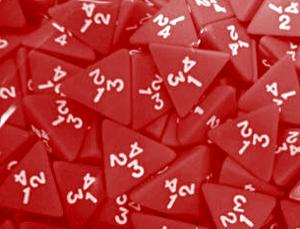A# tetrahedronClose packing of jumbled tetrahedra. Image: A. Jaoshvili, P. Chaikin, and A. Esakia.

A tetrahedron is a four-sided polyhedron. A regular tetrahedron, one of the Platonic solids, is a regular three-sided pyramid in which the base-edges and side-edges are of equal length. The projection of a regular tetrahedron can be an equilateral triangle or a square. The centers of the faces of a tetrahedron form another tetrahedron.

Research by Alexander Jaoshvili of New York University and colleagues, published in Physical Reviews Letters in 2010,1 showed that the tetrahedron is the most efficient shape for filling a container randomly with identically-shaped objects. They found that the tetrahedra were packed tightly enough to occupy 76% of their containers. In comparison, randomly packed spheres fill up to 64% of space, while the figure for ellipsoids can be as high as 74%.

### Reference

1. Jaoshvili, A., Esakia, A., Porrati, M., and Chaikin, P. M. "Experiments on the random packing of tetrahedral dice," Physical Review Letters, 104, 185501 (2010).

## De Malves' theorem

De Malves' theorem, or de Gua's theorem, is the three-dimensional equivalent of Pythagoras' theorem. It states that given a tetrahedron in which the edges meeting at one vertex, X, form three right angles (i.e. the tetrahedron is the result of chopping off the corner of a cuboid), the square of the face opposite X is equal to the sum of the squares of the other three faces. The theorem is named after the French mathematician Jean Paul de Gua de Malves (1713–1785).